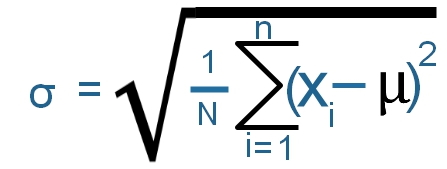# Standard Deviation σ1. Find out the average value (μ ) of the numbers or the list
2. Find out the sum (Σ) of square of difference between number and average value
3. Divide the sum by number of elements ( N )
4. Take the square root of the above division
We will create a Python function to return the Standard Deviation.
Note that this is Population Standard Deviation
``````import math
list1=[12,13,15,11,9,12,13,10,11,12,13,7,8]
def my_stddev(my_list):
my_sum=0
for i in my_list:
my_sum=my_sum+i
#print(my_sum)
print("Sum :",my_sum)
my_no=len(my_list)

my_avg=my_sum/my_no
print("Average",my_avg)
sum_avg=0.0
for i in my_list:
sum_avg=sum_avg+math.pow((i-my_avg),2)
my_variance = sum_avg/my_no
print("Variance :",my_variance )
std=math.sqrt(my_variance)
return std

print("Standard Deviation",my_stddev(list1))``````
Output
``````Sum : 146
Average 11.23076923076923
Variance : 4.6390532544378695
Standard Deviation 2.1538461538461537``````
We will compare the Standard Deviation values by using Pandas, Numpy and Python statistics library. Note the difference in values as there are two different formulas to get the Standard Deviation. The Population method uses N and Sample method uses N - 1, where N is the total number of elements.

## Using Pandas

Read more on Pandas here. We can use ddof option to tell Delta Degrees of Freedom. Default value of ddof is 1. The code with output is here.
``````list1=[12,13,15,11,9,12,13,10,11,12,13,7,8]
my_dict={'l1':list1}
my_data = pd.DataFrame(data=my_dict)
print(my_data['l1'].std(ddof=0))  # 2.1538461538461537
print(my_data['l1'].std(ddof=1))  # 2.24179415327122``````
Note that we are getting the same value in Python and Pandas by using `ddof=0` in Pandas std() function.

## Using Numpy

Read more on Numpy here. There is a built in standar deviation function in Numpy.
``````import numpy as np
my_data=np.array(list1)
print(my_data.std(ddof=0)) # 2.153846153846154
print(my_data.std(ddof=1)) # 2.2417941532712202``````
Here also we are getting same value as Python by using `ddof=0`

## Using statistics

We will use the statistics library
``````import statistics
print("Standard Deviation : ",statistics.stdev(list1))``````
Output is here
``Standard Deviation :  2.24179415327122``
The statistics library uses sample Standar Deviation.
Subscribe to our YouTube Channel here

## Subscribe

* indicates required
Subscribe to plus2netplus2net.com